## Points and Lines in the Plane

### Learning Outcomes

• Define the components of the Cartesian coordinate system.
• Plot points on the Cartesian coordinate plane.
• Use the distance formula to find the distance between two points in the plane.
• Use the midpoint formula to find the midpoint between two points.
• Plot linear equations in two variables on the coordinate plane.
• Use intercepts to plot lines.
• Use a graphing utility to graph a linear equation on a coordinate plane.

Tracie set out from Elmhurst, IL to go to Franklin Park. On the way, she made a few stops to do errands. Each stop is indicated by a red dot in the figure below. Laying a rectangular coordinate grid over the map, we can see that each stop aligns with an intersection of grid lines. In this section, we will learn how to use grid lines to describe locations and changes in locations.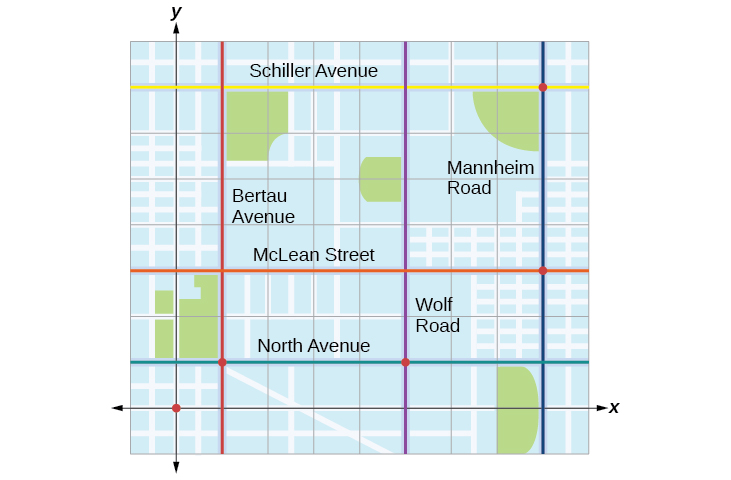## Plotting Points on the Coordinate Plane

An old story describes how seventeenth-century philosopher/mathematician René Descartes invented the system that has become the foundation of algebra while sick in bed. According to the story, Descartes was staring at a fly crawling on the ceiling when he realized that he could describe the fly’s location in relation to the perpendicular lines formed by the adjacent walls of his room. He viewed the perpendicular lines as horizontal and vertical axes. Further, by dividing each axis into equal unit lengths, Descartes saw that it was possible to locate any object in a two-dimensional plane using just two numbers—the displacement from the horizontal axis and the displacement from the vertical axis.

While there is evidence that ideas similar to Descartes’ grid system existed centuries earlier, it was Descartes who introduced the components that comprise the Cartesian coordinate system, a grid system having perpendicular axes. Descartes named the horizontal axis the x-axis and the vertical axis the y-axis.

The Cartesian coordinate system, also called the rectangular coordinate system, is based on a two-dimensional plane consisting of the x-axis and the y-axis. Perpendicular to each other, the axes divide the plane into four sections. Each section is called a quadrant; the quadrants are numbered counterclockwise as shown in the figure below.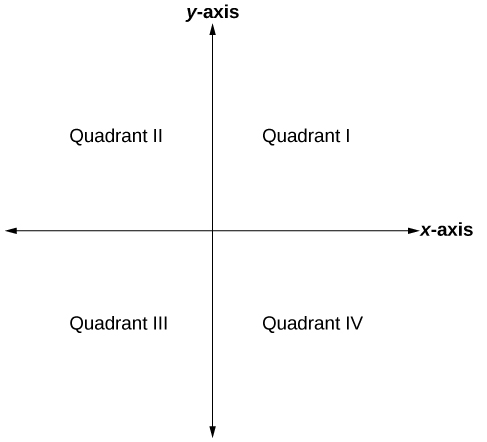The Cartesian coordinate system with all four quadrants labeled.

### Try It

The center of the plane is the point at which the two axes cross. It is known as the origin or point $\left(0,0\right)$. From the origin, each axis is further divided into equal units: increasing, positive numbers to the right on the x-axis and up the y-axis; decreasing, negative numbers to the left on the x-axis and down the y-axis. The axes extend to positive and negative infinity as shown by the arrowheads in the figure below.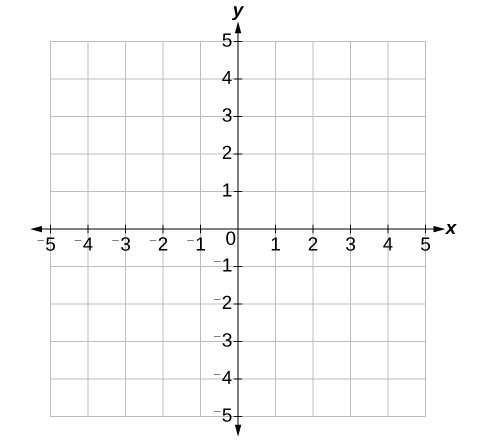Each point in the plane is identified by its x-coordinate, or horizontal displacement from the origin, and its y-coordinate, or vertical displacement from the origin. Together we write them as an ordered pair indicating the combined distance from the origin in the form $\left(x,y\right)$. An ordered pair is also known as a coordinate pair because it consists of and y-coordinates. For example, we can represent the point $\left(3,-1\right)$ in the plane by moving three units to the right of the origin in the horizontal direction and one unit down in the vertical direction.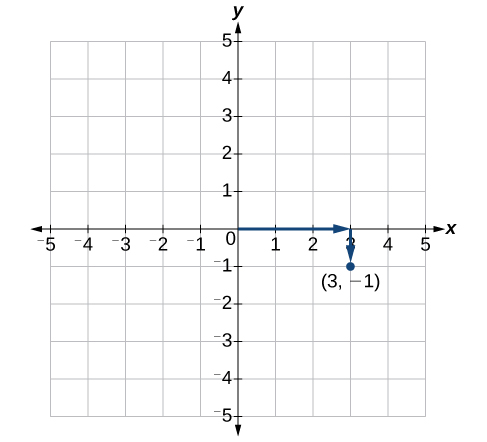An illustration of how to plot the point (3,-1).

When dividing the axes into equally spaced increments, note that the x-axis may be considered separately from the y-axis. In other words, while the x-axis may be divided and labeled according to consecutive integers, the y-axis may be divided and labeled by increments of 2 or 10 or 100. In fact, the axes may represent other units such as years against the balance in a savings account or quantity against cost. Consider the rectangular coordinate system primarily as a method for showing the relationship between two quantities.

### A General Note: Cartesian Coordinate System

A two-dimensional plane where the

• x-axis is the horizontal axis
• y-axis is the vertical axis

A point in the plane is defined as an ordered pair, $\left(x,y\right)$, such that x is determined by its horizontal distance from the origin and y is determined by its vertical distance from the origin.

### Example: Plotting Points in a Rectangular Coordinate System

Plot the points $\left(-2,4\right)$, $\left(3,3\right)$, and $\left(0,-3\right)$ in the coordinate plane.

## Distance in the Plane

Derived from the Pythagorean Theorem, the distance formula is used to find the distance between two points in the plane. The Pythagorean Theorem, ${a}^{2}+{b}^{2}={c}^{2}$, is based on a right triangle where a and b are the lengths of the legs adjacent to the right angle, and c is the length of the hypotenuse.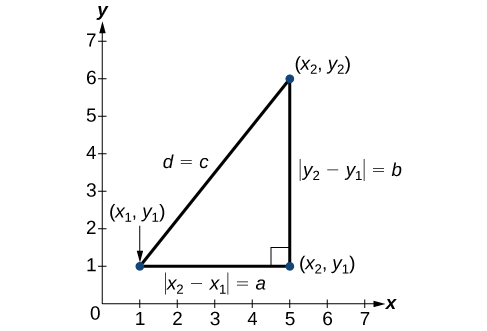The relationship of sides $|{x}_{2}-{x}_{1}|$ and $|{y}_{2}-{y}_{1}|$ to side d is the same as that of sides a and b to side c. We use the absolute value symbol to indicate that the length is a positive number because the absolute value of any number is positive. (For example, $|-3|=3$. ) The symbols $|{x}_{2}-{x}_{1}|$ and $|{y}_{2}-{y}_{1}|$ indicate that the lengths of the sides of the triangle are positive. To find the length c, take the square root of both sides of the Pythagorean Theorem.

${c}^{2}={a}^{2}+{b}^{2}\rightarrow c=\sqrt{{a}^{2}+{b}^{2}}$

It follows that the distance formula is given as

${d}^{2}={\left({x}_{2}-{x}_{1}\right)}^{2}+{\left({y}_{2}-{y}_{1}\right)}^{2}\to d=\sqrt{{\left({x}_{2}-{x}_{1}\right)}^{2}+{\left({y}_{2}-{y}_{1}\right)}^{2}}$

We do not have to use the absolute value symbols in this definition because any number squared is positive.

### A General Note: The Distance Formula

Given endpoints $\left({x}_{1},{y}_{1}\right)$ and $\left({x}_{2},{y}_{2}\right)$, the distance between two points is given by

$d=\sqrt{{\left({x}_{2}-{x}_{1}\right)}^{2}+{\left({y}_{2}-{y}_{1}\right)}^{2}}$

### Example: Finding the Distance between Two Points

Find the distance between the points $\left(-3,-1\right)$ and $\left(2,3\right)$.

### Try It

Find the distance between two points: $\left(1,4\right)$ and $\left(11,9\right)$.

### Example: Finding the Distance between Two Locations

Let’s return to the situation introduced at the beginning of this section.

Tracie set out from Elmhurst, IL to go to Franklin Park. On the way, she made a few stops to do errands. Each stop is indicated by a red dot. Find the total distance that Tracie traveled. Compare this with the distance between her starting and final positions.

### Using the Midpoint Formula

When the endpoints of a line segment are known, we can find the point midway between them. This point is known as the midpoint and the formula is known as the midpoint formula. Given the endpoints of a line segment, $\left({x}_{1},{y}_{1}\right)$ and $\left({x}_{2},{y}_{2}\right)$, the midpoint formula states how to find the coordinates of the midpoint $M$.

$M=\left(\frac{{x}_{1}+{x}_{2}}{2},\frac{{y}_{1}+{y}_{2}}{2}\right)$

A graphical view of a midpoint is shown below. Notice that the line segments on either side of the midpoint are congruent.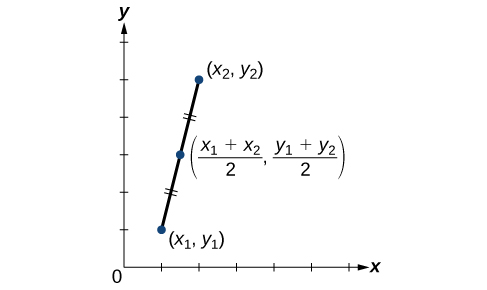### Example: Finding the Midpoint of a Line Segment

Find the midpoint of the line segment with the endpoints $\left(7,-2\right)$ and $\left(9,5\right)$.

### Try It

Find the midpoint of the line segment with endpoints $\left(-2,-1\right)$ and $\left(-8,6\right)$.

### Example: Finding the Center of a Circle

The diameter of a circle has endpoints $\left(-1,-4\right)$ and $\left(5,-4\right)$. Find the center of the circle.

## Graphing Linear Equations

We can plot a set of points to represent an equation. When such an equation contains both an x variable and a y variable, it is called an equation in two variables. Its graph is called a graph in two variables. Any graph on a two-dimensional plane is a graph in two variables.

Suppose we want to graph the equation $y=2x - 1$. We can begin by substituting a value for x into the equation and determining the resulting value of y. Each pair of and y-values is an ordered pair that can be plotted. The table below lists values of x from –3 to 3 and the resulting values for y.

 $x$ $y=2x - 1$ $\left(x,y\right)$ $-3$ $y=2\left(-3\right)-1=-7$ $\left(-3,-7\right)$ $-2$ $y=2\left(-2\right)-1=-5$ $\left(-2,-5\right)$ $-1$ $y=2\left(-1\right)-1=-3$ $\left(-1,-3\right)$ $0$ $y=2\left(0\right)-1=-1$ $\left(0,-1\right)$ $1$ $y=2\left(1\right)-1=1$ $\left(1,1\right)$ $2$ $y=2\left(2\right)-1=3$ $\left(2,3\right)$ $3$ $y=2\left(3\right)-1=5$ $\left(3,5\right)$

We can plot these points from the table. The points for this particular equation form a line, so we can connect them. This is not true for all equations.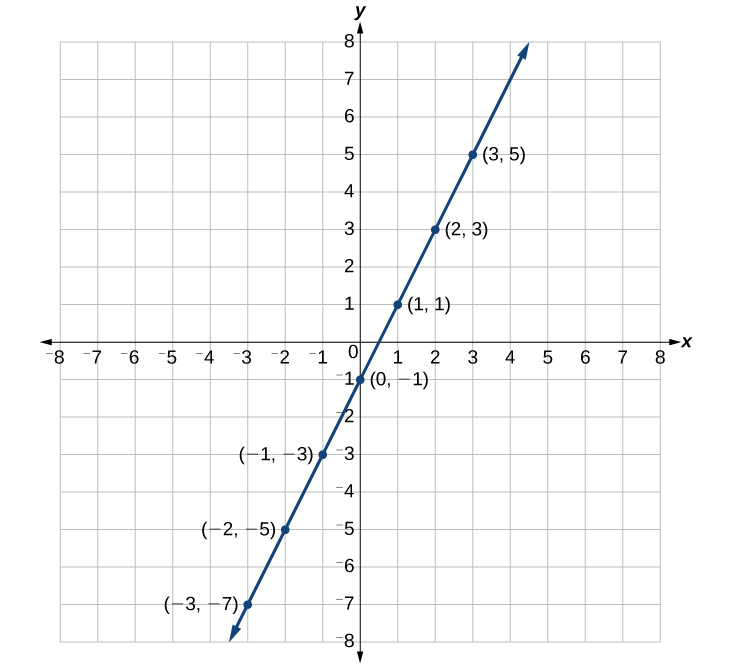Note that the x-values chosen are arbitrary regardless of the type of equation we are graphing. Of course, some situations may require particular values of x to be plotted in order to see a particular result. Otherwise, it is logical to choose values that can be calculated easily, and it is always a good idea to choose values that are both negative and positive. There is no rule dictating how many points to plot, although we need at least two to graph a line. Keep in mind, however, that the more points we plot, the more accurately we can sketch the graph.

### How To: Given an equation, graph by plotting points

1. Make a table with one column labeled x, a second column labeled with the equation, and a third column listing the resulting ordered pairs.
2. Enter x-values down the first column using positive and negative values. Selecting the x-values in numerical order will make graphing easier.
3. Select x-values that will yield y-values with little effort, preferably ones that can be calculated mentally.
4. Plot the ordered pairs.
5. Connect the points if they form a line.

### Example: Graphing an Equation in Two Variables by Plotting Points

Graph the equation $y=-x+2$ by plotting points.

### Try It

Construct a table and graph the equation by plotting points: $y=\frac{1}{2}x+2$.

### Using Intercepts to Plot Lines in the Coordinate Plane

The intercepts of a graph are points where the graph crosses the axes. The x-intercept is the point where the graph crosses the x-axis. At this point, the y-coordinate is zero. The y-intercept is the point where the graph crosses the y-axis. At this point, the x-coordinate is zero.

To determine the x-intercept, we set y equal to zero and solve for x. Similarly, to determine the y-intercept, we set x equal to zero and solve for y. For example, lets find the intercepts of the equation $y=3x - 1$.

To find the x-intercept, set $y=0$.

$\begin{array}{llllll}y=3x - 1\hfill & \hfill \\ 0=3x - 1\hfill & \hfill \\ 1=3x\hfill & \hfill \\ \frac{1}{3}=x\hfill & \hfill \\ \left(\frac{1}{3},0\right)\hfill & x\text{-intercept}\hfill \end{array}$

To find the y-intercept, set $x=0$.

$\begin{array}{lllll}y=3x - 1\hfill & \hfill \\ y=3\left(0\right)-1\hfill & \hfill \\ y=-1\hfill & \hfill \\ \left(0,-1\right)\hfill & y\text{-intercept}\hfill \end{array}$

We can confirm that our results make sense by observing a graph of the equation. Notice that the graph crosses the axes where we predicted it would.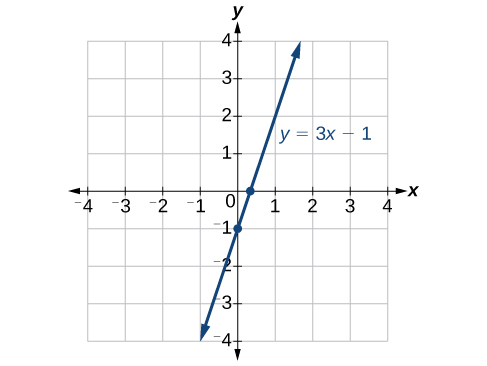### How To: Given an equation, find the intercepts

1. Find the x-intercept by setting $y=0$ and solving for $x$.
2. Find the y-intercept by setting $x=0$ and solving for $y$.

### Example: Finding the Intercepts of the Given Equation

Find the intercepts of the equation $y=-3x - 4$. Then sketch the graph using only the intercepts.

### Try It

Find the intercepts of the equation and sketch the graph: $y=-\frac{3}{4}x+3$.

### Using a Graphing Utility to Plot Lines

Most graphing calculators require similar techniques to graph an equation. The equations sometimes have to be manipulated so they are written in the style y=_____. The TI-84 Plus and many other calculator makes and models have a mode function, which allows the window (the screen for viewing the graph) to be altered so the pertinent parts of a graph can be seen.

For example, the equation $y=2x - 20$ has been entered in the TI-84 Plus as shown below. The resulting graph is shown. Notice that we cannot see on the screen where the graph crosses the axes. The standard window screen on the TI-84 Plus shows $-10\le x\le 10$, and $-10\le y\le 10$.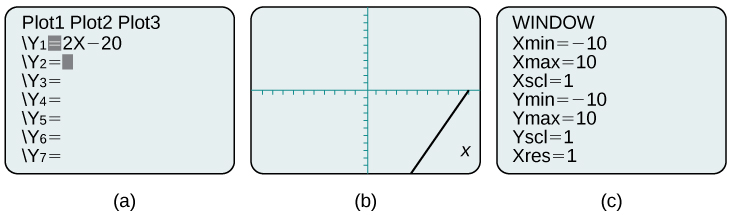(a) Enter the equation. (b) This is the graph in the original window. (c) These are the original settings.

By changing the window to show more of the positive x-axis and more of the negative y-axis, we have a much better view of the graph and the x and y-intercepts. See (a) and (b) below.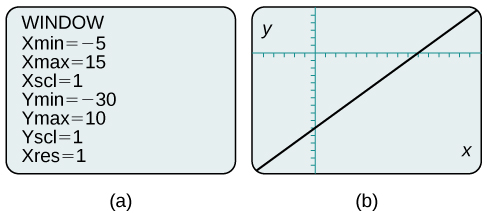(a) This screen shows the new window settings. (b) We can clearly view the intercepts in the new window.

### Example: Using a Graphing Utility to Graph an Equation

Use a graphing utility to graph the equation: $y=-\frac{2}{3}x-\frac{4}{3}$.

## Key Concepts

• We can locate or plot points in the Cartesian coordinate system using ordered pairs which are defined as displacement from the x-axis and displacement from the y-axis.
• An equation can be graphed in the plane by creating a table of values and plotting points.
• Using a graphing calculator or a computer program makes graphing equations faster and more accurate. Equations usually have to be entered in the form y=_____.
• Finding the x- and y-intercepts can define the graph of a line. These are the points where the graph crosses the axes.
• The distance formula is derived from the Pythagorean Theorem and is used to find the length of a line segment.
• The midpoint formula provides a method of finding the coordinates of the midpoint by dividing the sum of the x-coordinates and the sum of the y-coordinates of the endpoints by 2.

## Glossary

Cartesian coordinate system
a grid system designed with perpendicular axes invented by René Descartes
equation in two variables
a mathematical statement, typically written in x and y, in which two expressions are equal
distance formula
a formula that can be used to find the length of a line segment if the endpoints are known
graph in two variables
the graph of an equation in two variables, which is always shown in two variables in the two-dimensional plane
intercepts
the points at which the graph of an equation crosses the x-axis and the y-axis
midpoint formula
a formula to find the point that divides a line segment into two parts of equal length
ordered pair
a pair of numbers indicating horizontal displacement and vertical displacement from the origin; also known as a coordinate pair, $\left(x,y\right)$
origin
the point where the two axes cross in the center of the plane, described by the ordered pair $\left(0,0\right)$